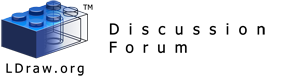Part authoring scripts and tools - Printable Version +- LDraw.org Discussion Forums (https://forums.ldraw.org) +-- Forum: LDraw Programs (https://forums.ldraw.org/forum-7.html) +--- Forum: Parts Author Tools (https://forums.ldraw.org/forum-24.html) +--- Thread: Part authoring scripts and tools (/thread-278.html) Part authoring scripts and tools - Tim Gould - 2011-08-03 Hi All, Sometimes I find it hard to keep track of all the little programs for part authoring: things like 'inliner' and Philo's myriad of tricky tools. Could we keep a master list of them in this thread? It also allows me (and others) to share scripts that we wouldn't upload to the master software list. As I will do in a few seconds. Tim Re: Part authoring scripts and tools - Tim Gould - 2011-08-03 This perl script creates a corner between two cylinders at arbitrary angle, segments and radius. It and the next script were used to make the tricky bits of this Code:```use Math::Trig; ## Computes the corner between two cylinders ## or radius \$rad at \$theta angle with \$segs segments \$pi=3.141592635; \$d2r=\$pi/180; ## Specify number of segments, angle, radius \$segs=16; #\$theta=55.238*\$d2r; \$theta=34.742*\$d2r; \$rad=4; ## Masks \$linemask="2 24  %.2f %.2f %.2f %.2f %.2f %.2f\n"; \$optmask="5 24  %.2f %.2f %.2f  %.2f %.2f %.2f  %.2f %.2f %.2f  %.2f %.2f %.2f\n"; \$trimask="3 16  %.2f %.2f %.2f  %.2f %.2f %.2f  %.2f %.2f %.2f\n"; \$quadmask="4 16  %.2f %.2f %.2f  %.2f %.2f %.2f  %.2f %.2f %.2f  %.2f %.2f %.2f\n"; # Internal variables \$thetah=\$theta/2; foreach \$n (0..(\$segs-1)) {     \$phi=\$n/\$segs*2*\$pi;     \$yeff=(1-cos(\$phi))*\$rad;     \$xeff=sin(\$phi)*\$rad;     \$x1=\$xeff;     \$y1=\$yeff;     \$z1=0;     \$x2=\$xeff;     \$y2=\$yeff;     \$z2=\$yeff*tan(\$thetah);     \$x3=\$xeff;     \$y3=\$yeff*cos(\$theta);     \$z3=\$yeff*sin(\$theta);     \$pts1->[\$n]=[\$x1,\$y1,\$z1];     \$pts2->[\$n]=[\$x2,\$y2,\$z2];     \$pts3->[\$n]=[\$x3,\$y3,\$z3]; } foreach \$n (0..(\$segs-1)) {     \$np=(\$n<(\$segs-1))?(\$n+1):0;     \$nm=(\$n>0)?(\$n-1):(\$segs-1);     \$x10=\$pts1->[\$n];     \$y10=\$pts1->[\$n];     \$z10=\$pts1->[\$n];     \$x20=\$pts2->[\$n];     \$y20=\$pts2->[\$n];     \$z20=\$pts2->[\$n];     \$x30=\$pts3->[\$n];     \$y30=\$pts3->[\$n];     \$z30=\$pts3->[\$n];     \$x1p=\$pts1->[\$np];     \$y1p=\$pts1->[\$np];     \$z1p=\$pts1->[\$np];     \$x2p=\$pts2->[\$np];     \$y2p=\$pts2->[\$np];     \$z2p=\$pts2->[\$np];     \$x3p=\$pts3->[\$np];     \$y3p=\$pts3->[\$np];     \$z3p=\$pts3->[\$np];     \$x1m=\$pts1->[\$nm];     \$y1m=\$pts1->[\$nm];     \$z1m=\$pts1->[\$nm];     \$x2m=\$pts2->[\$nm];     \$y2m=\$pts2->[\$nm];     \$z2m=\$pts2->[\$nm];     \$x3m=\$pts3->[\$nm];     \$y3m=\$pts3->[\$nm];     \$z3m=\$pts3->[\$nm];     printf(\$linemask,        \$x20, \$y20, \$z20,        \$x2p, \$y2p, \$z2p);     if ((\$x10!=\$x30) || (\$y10!=\$y30) || (\$z10!=\$z30)) {     printf(\$optmask,            \$x10, \$y10, \$z10, \$x20, \$y20, \$z20,            \$x1p, \$y1p, \$z1p, \$x1m, \$y1m, \$z1m);     printf(\$optmask,            \$x30, \$y30, \$z30, \$x20, \$y20, \$z20,            \$x3p, \$y3p, \$z3p, \$x3m, \$y3m, \$z3m);     printf(\$quadmask,            \$x1p,\$y1p,\$z1p,\$x10,\$y10,\$z10,            \$x20,\$y20,\$z20,\$x2p,\$y2p,\$z2p);     printf(\$quadmask,            \$x2p,\$y2p,\$z2p,\$x20,\$y20,\$z20,            \$x30,\$y30,\$z30,\$x3p,\$y3p,\$z3p);     }     else {     printf(\$trimask,            \$x1p,\$y1p,\$z1p,\$x10,\$y10,\$z10,            \$x2p,\$y2p,\$z2p);     printf(\$trimask,            \$x2p,\$y2p,\$z2p,            \$x30,\$y30,\$z30,\$x3p,\$y3p,\$z3p);     } }``` Re: Part authoring scripts and tools - Tim Gould - 2011-08-03 This perl script creates the intersection between a cylinder and a plane at a given radius, segments and angle Code:```use Math::Trig; ## Computes the intersection between a cylinder ## and the yz-plane. ## Radius \$rad at \$theta angle with \$segs segments \$pi=3.141592635; \$d2r=\$pi/180; ## Specify number of segments, angle, radius \$segs=16; #\$theta=34.742*\$d2r; \$theta=(90-34.742)*\$d2r; \$rad=4; ## Masks \$linemask="2 24  %.2f %.2f %.2f %.2f %.2f %.2f\n"; \$optmask="5 24  %.2f %.2f %.2f  %.2f %.2f %.2f  %.2f %.2f %.2f  %.2f %.2f %.2f\n"; \$trimask="3 16  %.2f %.2f %.2f  %.2f %.2f %.2f  %.2f %.2f %.2f\n"; \$quadmask="4 16  %.2f %.2f %.2f  %.2f %.2f %.2f  %.2f %.2f %.2f  %.2f %.2f %.2f\n"; foreach \$n (0..(\$segs-1)) {     \$phi=\$n/\$segs*2*\$pi;     \$yeff=(1-cos(\$phi))*\$rad;     \$xeff=sin(\$phi)*\$rad;     \$x1=\$xeff;     \$y1=\$yeff*sin(\$theta);     \$z1=\$yeff*cos(\$theta);     \$x2=\$xeff;     \$y2=0;     \$z2=\$yeff/cos(\$theta);     \$pts1->[\$n]=[\$x1,\$y1,\$z1];     \$pts2->[\$n]=[\$x2,\$y2,\$z2]; } foreach \$n (0..(\$segs-1)) {     \$np=(\$n<(\$segs-1))?(\$n+1):0;     \$nm=(\$n>0)?(\$n-1):(\$segs-1);     \$x10=\$pts1->[\$n];     \$y10=\$pts1->[\$n];     \$z10=\$pts1->[\$n];     \$x20=\$pts2->[\$n];     \$y20=\$pts2->[\$n];     \$z20=\$pts2->[\$n];     \$x1p=\$pts1->[\$np];     \$y1p=\$pts1->[\$np];     \$z1p=\$pts1->[\$np];     \$x2p=\$pts2->[\$np];     \$y2p=\$pts2->[\$np];     \$z2p=\$pts2->[\$np];     \$x1m=\$pts1->[\$nm];     \$y1m=\$pts1->[\$nm];     \$z1m=\$pts1->[\$nm];     \$x2m=\$pts2->[\$nm];     \$y2m=\$pts2->[\$nm];     \$z2m=\$pts2->[\$nm];     printf(\$linemask,        \$x20, \$y20, \$z20,        \$x2p, \$y2p, \$z2p);     if ((\$x10!=\$x30) || (\$y10!=\$y30) || (\$z10!=\$z30)) {     printf(\$optmask,            \$x10, \$y10, \$z10, \$x20, \$y20, \$z20,            \$x1p, \$y1p, \$z1p, \$x1m, \$y1m, \$z1m);     printf(\$quadmask,            \$x1p,\$y1p,\$z1p,\$x10,\$y10,\$z10,            \$x20,\$y20,\$z20,\$x2p,\$y2p,\$z2p);     }     else {     printf(\$trimask,            \$x1p,\$y1p,\$z1p,\$x10,\$y10,\$z10,            \$x2p,\$y2p,\$z2p);     } }``` Re: Part authoring scripts and tools - Jim DeVona - 2011-08-04 Here are some scripts I've written. They're not all necessarily part authoring tools, but if anyone else finds them useful I suspect it'd be the part authoring crowd. LDMerge - Command line script to combine LDraw directories. It keeps of record of changes which can be used to "undo" the merge. Primarily useful for temporarily merging unofficial parts with official library in order to use programs that don't recognize the /Unofficial directory. LDTrim - Command line utility to reformat LDraw code (trim whitespace, justify columns, strip extraneous zeros, etc). Most of this can be done with LDDP but it's handy to have options. "Export to LDraw" plugin for Google Sketchup - Derived from example by Jim Foltz. Re: Part authoring scripts and tools - Tim Gould - 2011-08-06 I'm an idiot and should have started this in the proper forum. Moving it now! Tim Re: Part authoring scripts and tools - Tore Eriksson - 2011-08-07 What do I need to run such scripts? Re: Part authoring scripts and tools - Tim Gould - 2011-08-07 Any standard installation of Perl should work. I use AcitvePerl on Windows and whatever is pre-installed on Linux. If you install ActivePerl just copy the code into a text file and give it a .pl extension (or drop me an email for the script). It should then run from the command line if your installation is smooth. The advantage is that it's totally portable, very quick to write, and easy to adapt. The disadvantage is that it's hard to read! Tim Re: Part authoring scripts and tools - Tore Eriksson - 2011-08-07 Thanks, Tim. I may give it a try. /Tore